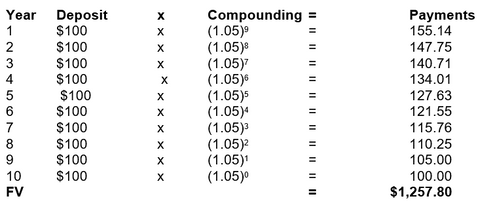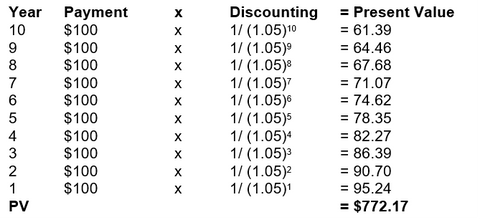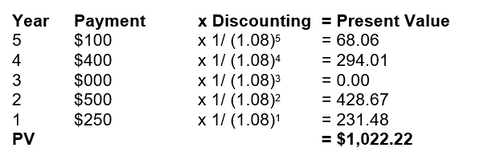Welcome to our website!

# Time Value of Money: Future Value, Present Value, Annuity, Perpetuity

Overview

We know that money has two general purposes: it serves as a store of value and as a medium of exchange. The term time value of money refers to all aspects of converting the value of cash flows at one point of time to their equivalent values at another time. It is important that we know the value of current and future cash flows to facilitate the financial planning process.

Check our model template here

Future Value

The term future value (FV) refers to the value of a cash flow at a specified rate of interest at the end of a stated length of time. A cash flow that is received today is worth more than the same amount received in the future. Cash in hand today may be invested to earn a return over a period.

For example, an investor receiving \$100 today may have the opportunity to invest the \$100 for one year at 10% per annum (p.a.). With a risk-free investment in an environment free from inflation, the investor will have \$110 value at the end of the one year. The principal plus the return on the investment over time represents the future value of the investment. Future value is the worth of an investment at a specified rate of interest at the end of a stated period.

To illustrate, suppose that we invest \$500 in an account earning 12% annual interest. At the end of one year, the future value of our investment is:

FV1 = (\$500) (1 + 0.12)

FV1 = (\$500) (1.12)

FV1 = \$560

If we leave the \$560 dollars in the account for another year, at the same rate, the future value is:

FV1 = (\$560) (1 + 0.12)

FV1 = (\$560) (1.12)

FV1 = \$627.20

We can arrive at the same result by using the future value formula with a two-year time frame.

FV2 = (\$500) (1 + 0.12)2

FV2 = (\$500) (1.2544)

FV2 = \$627.20

Check our model template here

Present Value

Discounting is the process we use to equate a future cash flow to its present value (PV). The calculation is the reversal of the compounding process.

The discount rate represents the opportunity cost of money, which is the rate of return that could have been earned on the best alternative investment. In finance, analysts often use the concept of opportunity cost to evaluate investment alternatives. The logic is to estimate the next best return scenario if a different investment decision had been made. Applying this concept to discount rates, analysts say that, given approximately equal time frames and levels of risk, the appropriate rate to discount cash flows is the rate of return that represents the next best alternative in the list of investment choices. The discount rate or opportunity cost is also called the investor's required rate of return.

For example, the \$1,000 cash flow expected in three years, given an annual discount rate of 10%, equals \$751.31 today. An investor with opportunities to invest at 10% compounded annually would be indifferent between receiving \$751.31 today or \$1,000 in three years, all other factors being equal.

PV = \$1,000 [ 1 / (1 + 0.10) ]3

PV = \$1,000 [0.75131]

PV = \$751.31

The zero-coupon bond concept has a very practical application in the valuing of securities. For example, it is a security that pays no interest; it is priced at a discount from the face value to give the investor a specific rate of return. The face value is the amount the issuer will pay at the time of maturity. The issuer prices the bond at a discount so that investors receive a return on their investments.

Suppose that a company is issuing zero-coupon bonds to raise funds for its operation. The bonds have a face value of \$10,000 and a maturity of one year. What would an investor requiring a 9% return be willing to pay for one of the bonds today?

PV = \$10,000 [ 1 / (1 + 0.09) ]1

PV = \$10,000 [ 0.917431]

PV = \$9,174.31

Check our model template here

Annuities

Many financial market securities involve more than one cash flow. An annuity is a security with a series of equal payments (or receipts) spaced evenly over a determined period.

Calculating the future value of an annuity is a straight-forward exercise. However, it can be tedious as the following example illustrates. Suppose that an investor invests \$100 each December 31st for the next ten years. The account pays an annual interest rate of 5%. What will be the value of the account at the end of the ten years?As you can see from the table, the interest on the deposit at the end of Year One is compounded for nine years, interest on the deposit at the end of Year Two for eight years, and so forth. The deposit at the end of Year Ten earns no interest. Thus, at the end of ten years, the total future value of this annuity is \$1,257.80.

You can use our file to see the formulas in excel required to perform this type of calculation here

The calculation of the present value of an annuity is the reversal of the compounding process for the future value. The idea is to discount each individual payment from the time of its expected receipt to the present. We can illustrate by building another table to calculate the present value of the annuity, ten payments of \$100, made on December 31st of each year, discounted at a rate of 5%.The present value of this annuity is \$772.17. This means that an investor with opportunities to invest in other investments of equal risk at 5% would be willing to pay \$772.17 for this annuity. Notice that because the payments are received at the end of each period, we discount them for the entire period.

You can use our file to see the formulas in excel required to perform this type of calculation here

Perpetuities

A perpetuity is special type of annuity where the time for the payments is infinity. Some examples of perpetuities are payments from an education endowment fund, or the dividends paid on preferred stock.

The future value of a perpetuity is always infinity and, therefore, pointless to calculate. The present value of a perpetuity is easily calculated. Let's use the formula with an example. An investor requires 16% return on investment. What will the investor be willing to pay for a share of preferred stock with a \$10 annual dividend?

PVp = \$10 x (1 / 0.16)

PVp = \$62.50

If the investor pays \$62.50 per share, she will receive a \$10 dividend per year, which equals a 16% return on her investment.

Uneven Payment Streams

Sometimes an investment has payments of unequal size or spacing. The present and future value formulas can be used to accommodate these irregularities. For instance, suppose that you deposit \$100 in an investment at the end of Year One, \$350 at the end of Year Three, \$250 at the end of Year Four, and \$200 at the end of Year Five. If the investment earns 6% compounded annually, what is the value (future value of unequal payments) of this investment at the end of Year Six?You can see that the first deposit is in the account for five years, earning compounded interest of \$33.82 during that time; no deposit is made in the second year; the third deposit earns compound interest for three years in the amount of \$66.86; and so on. The total interest the account will earn for the six years is \$1,043.58 – \$900.00 (the total amount of all the deposits) = \$143.58.

Regarding the present value of unequal payments, the idea is to discount each payment or cash flow from the time of receipt back to the present time. The present value is the sum of all the discounted cash flows.

To illustrate, we will calculate the present value of an investment with expected payments of \$250 at the end of Year One, \$500 at the end of Year Two, \$400 at the end of Year Four, and \$100 at the end of Year Five. The investor's required rate of return is 8%.The present value of this investment is \$1,022.22. Another approach to solving this problem is to use our model template with ready made excel formulas. You can download the file here.

Summary

The future value of money at the end of a defined period results from adding the amount of interest that may be earned on an investment to the principal amount.

Sometimes we want to know the current value of a cash flow that will occur at some future date. Discounting is the process for equating a future cash flow with its present value. The discount rate may be referred to as the opportunity cost of an investment or the investor's required rate of return.

An annuity is a security that involves a series of equal payments made at regular time intervals. To derive the future value of an annuity, each payment is compounded for the remaining life of the security. Calculating the present value of an annuity is the reverse process of compounding.

A perpetuity is a special type of annuity with an infinite number of payment periods. The future value of a perpetuity is infinite — and of little consequence. The present value is easy to calculate and provides the investor with information to facilitate a comparison of investment prices.

The present and future values of investments with payments of uneven size or spacing also may be calculated by totaling the compounded or discounted values of the cash flows.

You can see that the present / future value of an investment depends on the discount / interest rate, the number of payments, the length of time until maturity, and the amount of each cash flow. By manipulating these factors, we can discover the future value of an interest-bearing investment or the discounted present value of future cash flows.

Check our model template related to the time value of money, bond and stock valuation here